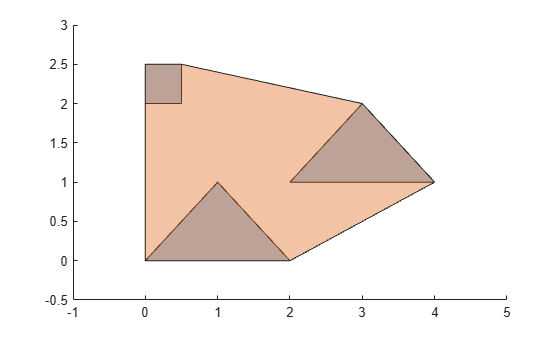# convhull

Convex hull of `polyshape`

## Syntax

``polyout = convhull(polyin)``

## Description

example

````polyout = convhull(polyin)` returns the convex hull of a `polyshape` object. `polyout` is a `polyshape` object or an array of `polyshape` objects the same size as `polyin`.```

## Examples

collapse all

Compute the convex hull of a polygon containing three solid regions.

```x1 = [0 1 2]; y1 = [0 1 0]; x2 = [2 3 4]; y2 = [1 2 1]; x3 = [0 0.5 0.5 0]; y3 = [2 2 2.5 2.5]; polyin = polyshape({x1,x2,x3},{y1,y2,y3}); plot(polyin) hold on polyout = convhull(polyin); plot(polyout)```## Input Arguments

collapse all

Input `polyshape`, specified as a scalar, vector, matrix, or multidimensional array.

Data Types: `polyshape`